# Integrals & the Area Under the Curve Flashcards

Integrals & the Area Under the Curve Flashcards
1/20 (missed) 0 0
Create Your Account To Continue Studying

As a member, you'll also get unlimited access to over 75,000 lessons in math, English, science, history, and more. Plus, get practice tests, quizzes, and personalized coaching to help you succeed.

Try it risk-free for 30 days. Cancel anytime.
###### Already registered? Login here for access
Additive Property of Integrals
The additive property is defined as: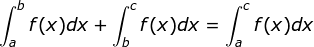Got it
Trapezoid Rule
A method to solve the area under a curve (the integral of a function) that uses the area of trapezoids drawn by the points on the graph.
Got it
Riemann sum
A method used to approximate the area under a curve (also known as an integral) that utilizes the area of rectangles to estimate the solution.
Got it
Right-handed Riemann sum
This Riemann sum calculation is solved using the height at the right points of the specific interval to estimate the area under a curve.
Got it
Using an interval of 1 from x = 0 to x = 5, find the value of the right-hand Riemann sum for the integral shown.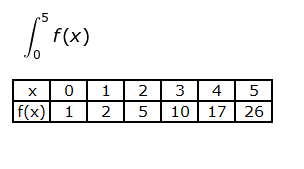60. The right-handed Riemann sum leaves us with 2+5+10+17+26, which equals 60.
Got it
Left-sided Riemann sum
Riemann sum calculation where the height at the left point of the specific interval is used to estimate the area under a curve
Got it
Estimate the integral of y = 2x from x = 0 to x = 5 using left Riemann sums. Use a delta x of 1.
20. On the interval from x = 0 to x = 5, we get 0+2+4+6+8, which equals 20.
Got it
Mid-point Riemann sum
Riemann sum type that uses the height at the middle point of a specific interval to estimate the area under a curve
Got it
Riemann sums are used to estimate the _____ under a curve.
area - Riemann sums are used as an estimation tool to approximate the area under a curve (also known as the integral).
Got it
Calculate the following: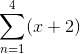The summation is 18
Got it

### Ready to move on?

or choose a specific lesson: See all lessons in this chapter
20 cards in set

## Flashcard Content Overview

This flashcard set will help you with solving integral problems that you may encounter in your calculus class. Cards in this set include flashcards on Riemann sums, the trapezoid rule, optimization problems, and definite and indefinite integrals. Use this set to prep for your next calculus test or to simply brush up on these concepts.

Front
Back
Calculate the following:The summation is 18
Riemann sums are used to estimate the _____ under a curve.
area - Riemann sums are used as an estimation tool to approximate the area under a curve (also known as the integral).
Mid-point Riemann sum
Riemann sum type that uses the height at the middle point of a specific interval to estimate the area under a curve
Estimate the integral of y = 2x from x = 0 to x = 5 using left Riemann sums. Use a delta x of 1.
20. On the interval from x = 0 to x = 5, we get 0+2+4+6+8, which equals 20.
Left-sided Riemann sum
Riemann sum calculation where the height at the left point of the specific interval is used to estimate the area under a curve
Using an interval of 1 from x = 0 to x = 5, find the value of the right-hand Riemann sum for the integral shown.60. The right-handed Riemann sum leaves us with 2+5+10+17+26, which equals 60.
Right-handed Riemann sum
This Riemann sum calculation is solved using the height at the right points of the specific interval to estimate the area under a curve.
Riemann sum
A method used to approximate the area under a curve (also known as an integral) that utilizes the area of rectangles to estimate the solution.
Trapezoid Rule
A method to solve the area under a curve (the integral of a function) that uses the area of trapezoids drawn by the points on the graph.
Additive Property of Integrals
The additive property is defined as:Zero Integral Property
This integral property is defined as: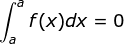Evaluate the following integral: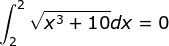The integral equals 0 because of the Zero Integral Property
Solve the integral: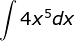The solution is: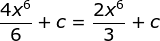Complete the following integral formula: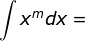The formula equals the following when m is not equal to -1: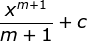The integral of acceleration with respect to time equals:
The velocity. Similarly, the integral of velocity with respect to time equals the speed.
Find y if y ' = 4x and y(0) = 4
y = 2x2 + 4
Sums Property of Integrals
The sums property is: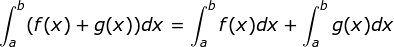Solve the following: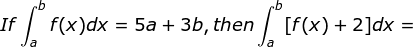3a + 5b
Use the following graph to solve g(0) + g'(0) + g''(0)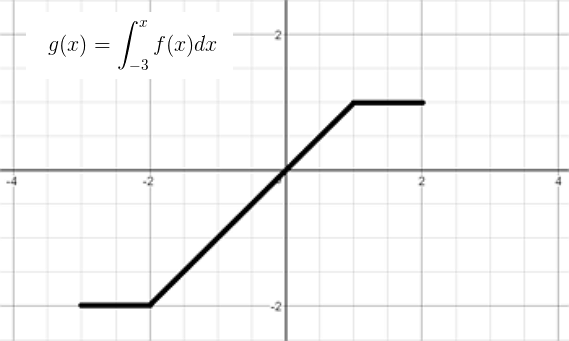The solution is -3.

Using the fundamental theorem of calculus, g(x) = (1/2)x2 - 4

g(0) = -4, g'(0) = 0, g''(0) = 1

Fundamental Theorem of Calculus
If f(x) is continuous from a to b, then: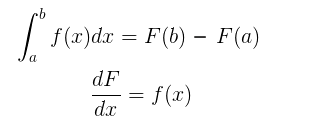To unlock this flashcard set you must be a Study.com Member.